Courses

# General Aspects of CMOS Technology (Part - 2) Electrical Engineering (EE) Notes | EduRev

## VLSI System Design

Created by: Machine Experts

## Electrical Engineering (EE) : General Aspects of CMOS Technology (Part - 2) Electrical Engineering (EE) Notes | EduRev

The document General Aspects of CMOS Technology (Part - 2) Electrical Engineering (EE) Notes | EduRev is a part of the Electrical Engineering (EE) Course VLSI System Design.
All you need of Electrical Engineering (EE) at this link: Electrical Engineering (EE)

Objectives

In this course you will learn the following

• Why polysilicon prefered over aluminium as gate material?
• Channel stopper Implant
• Local Oxidation of silicon (LOCOS)

11. Why polysilicon prefered over aluminium as gate material?

Because-

1) Penetration of silicon substrate: If aluminium metal is deposited as gate, we can't increase the temperature beyond 500 degree celcius due to the fact that aluminium will then start penetrating the silicon substrate and act as p-type impurity.

2) Problem with non-self alignment: In case of aluminium gate, we have to first create source and drain and then gate implant. We can't do the reverse because diffusion is a high temperature process. And this creates parasitic overlap input capacitances Cgd and Cgs(figure 11.11).Cgd is more harmful because it is a feedback capacitance and hence it is reflected on the input magnified by (k+1) times (recall Miller's theorem), where is the gain. So if aluminum is used, the input capacitance increases unnecessarily which further increases the charging time of the input capacitance. Therefore output doesn't appear immediately.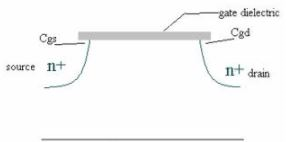Figure 11.11: Self-alignment is not possible in case of Al gate due to Cgd and Cgs

If poly-silicon is used instead, it is possible to first create gate and then source & drain implant, which eliminates the problem of overlap capacitances Cgd and Cgs.

Resistivity of poly-silicon is 10^8 ohm/cm. So we need to dope poly-silicon so that it resembles a metal like Al and its resistance is reduced to 100 or 300 ohm (although its still greater than Al).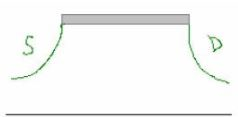Figure 11.12 Self-alignment possible in case of poly-silicon

Time for charging capacitance varies as negative exponential of (RC)^(-1) where and are resistance and capacitance of the device. As we know the resistance is directly propotional to the length, so poly-silicon length should be kept small so that the resistance is not large, otherwise the whole purpose of decreasing C (hence the time constant RC) will be nullified.

11.2 Channel stopper Implant

As we know millions of transistors are fabricated on a single chip. To seperate (insulate) these from each-other, we grow thick oxides (called field oxides). So, at very high voltages, inversion may set in the region below the field oxide also, despite the large thickness of these oxides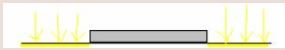Figure 11.21: channel stopper implant before field oxide region is grown (yellow color region)

To avoid this problem, we do an implant in this region before growing the field oxide layer so that threshold voltage for this region is much greater than that for the desired active transistor channel region. This implant layer is called channel stopper implant. (as shown in figure 11.21)

11.3 Local Oxidation of Silicon (LOCOS)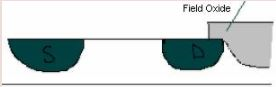Figure 11.31: Formation of LOCOS

Creation of LOCOS:

During etching, anything irregular becomes more irregular. So we grow oxide fields 50% above and 50% below the wafer. This is called LOCal Oxidation of Silicon(LOCOS).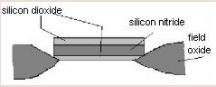Figure 11.32: bird's beak

0.45 mU of silicon, when oxidized, becomes 1 mU of SiO2 because of change in density. When field oxides are grown, there is an encroachment of the oxide layer in the active transistor region below the gate oxide, because of the affinity of the SiO2 gate oxide for oxygen. The resulting structure resembles a bird's beak (as shown in figure 11.32) . This affects the device performance.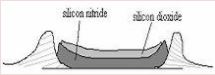Figure 11.33: bird's creast

If we use Si3N4 as the gate dielectric, it will not let oxygen pass through. But due to mismatch of the thermal coefficients of Si and Si3N4, hence the resulting stress produces a non-planar structure called bird's crest(as shown in figure 11.33) .

The thermal coefficients of Si and SiO2 match. So when Si3N4 is used as the gate dielectric, we first grow a thin oxide layer underneath. The stress, which would otherwise be generated on the account of the difference in the thermal coefficients of Siand SiO2 is now reduced. Since SiO2 is now there, bird's beak will be formed.

Offer running on EduRev: Apply code STAYHOME200 to get INR 200 off on our premium plan EduRev Infinity!

,

,

,

,

,

,

,

,

,

,

,

,

,

,

,

,

,

,

,

,

,

;